## Introduction

This repository is a single point of reference for the TFxIDF weighting equations and is meant to be a guide for the student and practitioner interested in building her own search engine. The acronym TF stands for 'term frequency' and IDF stands for 'inverse document frequency', and these two numbers form the basis of computing the similarity between two pieces of text. The weighting equation is explained in detail in subsequent sections. Over the years the equation has morphed into sophisticated forms and a myriad variety is in use in systems and research papers.

What is problematic is authors of research papers and system builders often name their abstractions (algorithms, classes, functions) TFxIDF without being explicit about its form in documentation. This kind of obfuscation makes it hard to reproduce experiments reported in research papers or make sense of the behavior of off-the-shelf search engines, leaving readers and practitioners with little to learn from. This repository is therefore meant to be a resource to point to when using TFxIDF in your work and avoid any kind of ambiguity about its meaning.

## Term Weights

#### I - A Vector Space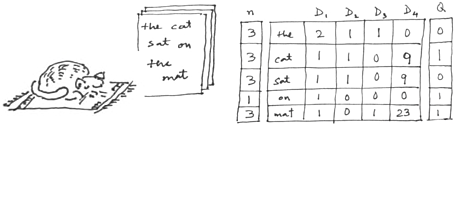The vector space model treats documents as vectors in t-dimensional space, where t is the size of the vocabulary (that is, the number of unique terms) of the collection of documents your system is searching in. The t components of each document vector is assigned a number that quantifies the importance of a term in that document. The components corresponding to terms not in the document is assigned zero. The simplest number to use in a component is a term's frequency in the document, say, f. More sophisticated forms would use some function of f, tf(f).

Having framed the entire collection into a t x N table (t rows for t terms in the vocabulary, and N columns for N documents in the collection), one other useful number that can be gleaned is the document frequency, n, of a term, or the number of documents the term is in. This is a measure of a term's global importance and formulated as some function df(n) just as tf(f) measures a term's local importance.

Table I above is an example showing a collection of 4 documents (about a cat on a mat) mapped into a vector space. The body of the table is populated with the term-frequencies or f-values, a column of document-frequencies or n-values is on the left and the documents are labeled D1 to D4. Q is the search query that reads 'cat on mat', also set in the vector space. For this collection, N = 4 and t = 5.

#### II - IDF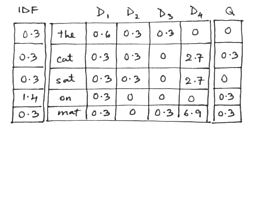It is logical to modulate the term's local importance tf(f) with the term's global importance df(n). If df(n) is log(N/n), something that varies inversely as n, it captures the term's importance in the entire collection. This form is called the 'inverse document frequency' (IDF). IDF is close to zero for a term that occurs in almost all documents and large if it occurs in just a few documents.

The weight of a term, w, or the TFxIDF equation, then takes this form:

``````w = tf(f) * df(n)
``````

In table II the n-value column is changed to df(n) (log(N/n)) and the f-values in the body replaced by w. The query's components in the column on the right undergo the identical transformations.

#### III - Length Normalization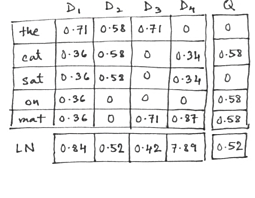Since we are dealing with vectors, it's possible to convert them to unit vectors. Logically this has the advantage of normalizing the documents' lengths. If a document like D4 has parts of it repeated, with no new information, you wouldn't want it to be any more 'relevant' than, say, D1. So unit document vectors and unit query vectors are used for the dot product and this is one kind of 'document length normalization' (g()). Adding this piece transforms the equation to:

``````            tf(f) * df(n)
w' = ----------------------------
sqrt(sum({tf(f) * df(n)}^2))
``````

The denominator is the Euclidean length of the vector; the square root of the sum of squares of the weights of the components of the vector.

Table III has a new row at the bottom, labeled LN, with the Euclidean lengths of each document vector (columns) and the w-values in the body replaced by w'-values.

#### IV & V - Ranking Search Results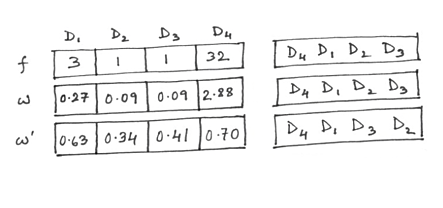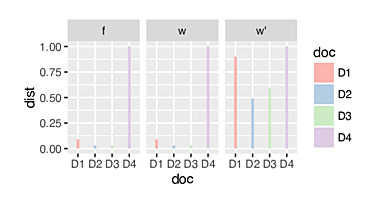The query's similarity to a document is obtained by computing the vector dot product between it and a document vector (columns in the tables). A dot product yields a single number or score, so now you have some numerical sense of 'similarity'. Then, arranging the N scores in descending order (ranked) is supposed to have ordered the documents in decreasing 'magnitude' of 'relevance' to the query. The score is represented as:

``````score(D,Q) = sum(wd' * wq')
``````

wd' and wq' is the w'-value of each component of the document vector and query vector respectively. The sum() is arithmetic sum over all the components of a vector.

Figure IV gathers the dot product values between the query Q and each of document D1 to D4. The three rows labeled f, w and w' show how the values change as we introduce IDF and LN one by one to the term-weight computation. The graph in figure V is meant for a relative comparison of the absolute values of the dot products on a scale of 0 to 1 within each type of weighting scheme (f, w, w'). D4 comes up trumps in all three sets just because of the preponderance of repeated terms in it. The weighting scheme w doesn't change relative positions in any way because all w-values were obtained by multiplying all f-values with a positive constant, the IDF. However, w' seems to change the game for D1, D2 and D3. Their scores jump up (relative to D4's score) and D1 is now in contention for the top spot. Normalizing for length prevents D4 from getting too far ahead. D2 and D3's tie is also broken.

## Vector Spaces & The SMART System

SMART was one of the earliest text search engines used for experiments in text-search based on the TFxIDF scheme described so far. SMART's similarity score computation is the same expression for score(D,Q) described earlier:

``````score(D,Q) = sum(wd' * wq')
``````

``````                          tf(f) * df(n)                 tf(qf) * df(n)
score(D,Q) = sum( ---------------------------- * ----------------------------- )
sqrt(sum({tf(f) * df(n)}^2))   sqrt(sum({tf(qf) * df(n)}^2))
``````

The outer sum() is over the matching terms of the document and query vector and the two inner sum() computations is over the t components of the t-dimensional vector space. qf is the frequency of the term in the query. The left half of the right-hand-side of the equation inside the outer sum() is the w' computation of the term in the document (i.e. wd') and the right half is its counterpart in the query (i.e. wq').

However, to make the computation more efficient, the expression was shortened by dropping the df(n) computation in wd'. This is because, a second pass through the t x N table would otherwise have been needed to modulate the tf(f) using the df(n) factor:

``````                         tf(f)               tf(qf) * df(n)
score(D,Q) = sum( ----------------- * ----------------------------- )
sqrt(sum(tf(f)^2))  sqrt(sum({tf(qf) * df(n)}^2))
``````

The length normalization factor shown so far was the Euclidean length of the vector. Over time several variations of this factor emerged. This generalized expression for the score subsumes this fact:

``````                  tf(f)     tf(qf) * df(n)
score(D,Q) = sum( ------ * --------------- )
g(G,L)        g(G,L)
``````

Where g(G,L) is a length normalization factor expressed as a function of some global collection statistics, G, and local document statistics L.

The authors of SMART had also devised a notation to represent the variations of weighting equations of this kind. Since the equation has three pieces each for a weight of term in the document and in the query, two three-letter pairs would denote the entire equation.

Through eight years of experimentation using these TFxIDF variants (TREC1-8), the most effective form was found to be a dtb.nnn. This is what it reads and nomenclature is explained in detail in the section to follow:

``````                  1+log(1+log(f))) * df(n)   qf * 1
score(D,Q) = sum( ------------------------ * ------ )
1 - s + s * b / avgb        1
``````

#### SMART's Term Weighting Triple Notation

The mnemonic used to describe TFxIDF weighting equations is:

``````xxx.xxx
``````

Of the two triples, the first represents, respectively, the term frequency (TF), document frequency (IDF), and vector length normalization factors (LN) for document terms, and the second triple denotes the same three things for query terms.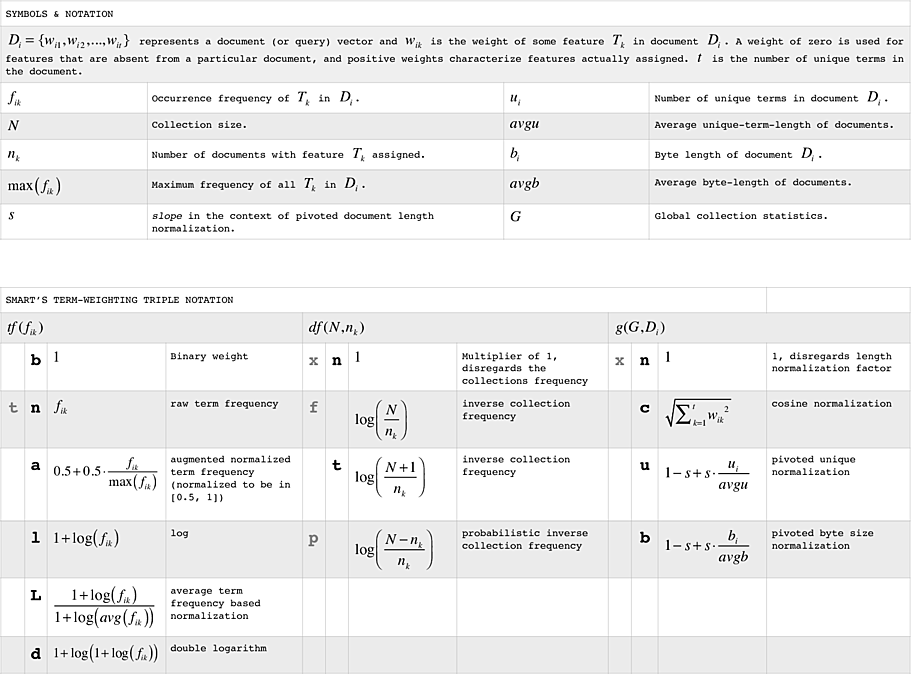• The letters in gray are is the scheme used in Salton's 1988 paper titled 'Term-weighting approaches in automatic text retrieval'. They were replaced with a new scheme in experiments reported thereafter.

#### Evolution of SMART'S tf-idf Equations

1. Salton G, Buckley C. Term-weighting approaches in automatic text retrieval. Information processing & management. 1988 Dec 31;24(5):513-23.
The effective schemes for:
document: tfc, nfc (or, p instead of f)
query: nfx, tfx, bfx (or p instead of f)

2. Buckley C. The importance of proper weighting methods. InProceedings of the workshop on Human Language Technology 1993 Mar 21 (pp. 349-352). Association for Computational Linguistics.

lnc.ltc is described as 'best' and ntc.ntc as 'most generally used' at that time.

3. Buckley, C., Allan, J. and Salton, G., 1993, March. Automatic retrieval with locality information using SMART. In Proceedings of the First Text REtrieval Conference TREC-1 (pp. 59-72).

On page 70 in the section titled 'Alternative Weighting Schemes' is the mention of not using the IDF factor to modulate the weights of terms in documents. The effect of this tweak is described as 'very reasonable, just a bit less effective'. The observations are:

nnc document weighting takes half the time to compute.
ntc document weighting is better in the case of feedback.
lnc document weighting is convenient as it avoids two passes.
ltc query weight is not generally recommended.
lnc.ltc > nnc.ntc by 20% of which 10% is due to ltc query weights and the other half is due to the lnc document weights. The improvement due to ltc query weights is almost certainly an artifact of TREC queries and possibly even an artifact of the second query set (queries 51-100).

4. Singhal A, Buckley C, Mitra M. Pivoted document length normalization. InProceedings of the 19th annual international ACM SIGIR conference on Research and development in information retrieval 1996 Aug 18 (pp. 21-29). ACM.

Reports use of Lnu document weights also termed as 'pivoted unique normalization' and lnb.ltb for degraded text collections. Value of slope, s = 0.2, and the 'pivot' was either the 'average unique-length' or 'average byte-length'. Section 4 mentions a reason for the shift from cosine normalization to u. Section 5 mentions a reason for the shift from max(tf) to L.

5. Singhal A, Choi J, Hindle D, Lewis DD, Pereira F. At&t at TREC-7. NIST SPECIAL PUBLICATION SP. 1999 Jul 7:239-52.

Reports use of dnb.dtn as baseline and dtb.dtn for query expansion. Note that, sometime between 1996 and 1999, avg(tf) was dropped [Citation?]. Here is an excerpt as the argument for using logarithm to modulate tf: "The main reasoning for use of a logarithmic tf-factor instead of (say) the raw tf of the terms is to ensure that high tf for one query term in a document does not place that document ahead of the other documents which have multiple query terms but with low tf values. By using a logarithmic tf-factor, the effect of tf dampens with increasing tf values, and single match usually doesn’t outweigh multiple matches."

6. Singhal A. Modern information retrieval: A brief overview. IEEE Data Eng. Bull.. 2001 Dec;24(4):35-43.

Mentions SMART's dtb.nnn and Okapi's BM25 as 'state-of-the art'. There is a typographic error in the BM25 formula; k1 in the denominator should be outside the bracket.

## From Probability & The Okapi System

A class of term-weighting equations were developed during the same time by another research group experimenting with the Okapi retrieval system. The form of the equations were derived from a probabilistic model that made use of a 2-Poisson distribution. This model was meant to provide the theoretical framework for the notion of 'similarity' between two text documents. Their formulation of the IDF factor too was grounded in a mathematically derivable theory. They reported experiments in TREC1 using the IDF factor only:

``````w = df(n)
``````

By TREC2 the variants proliferated (BM0, BM1, BM11, BM15; BM for 'Best Match') and the equation lengthened considerably. S was a scaling factor and C a correction factor that compensated for variations in document lengths. C was dropped eventually when the document-length normalization was weaved into the transformation tf().

``````w = S * tf(f) * df(n) * qtf(qf) + C
``````

TREC3 then introduced the BM25 weighting equation. It had the same structure, and various forms of tf(), df() and qtf() were used in experimentation over the next five years (TREC4-8). The score between a document and query, in this scheme can be generalized as:

``````score(D,Q) = sum(w)
``````

The sum() is the arithmetic sum over the terms in the query that are also in the document, i.e. the matching terms.

#### The BMxx Weighting Equations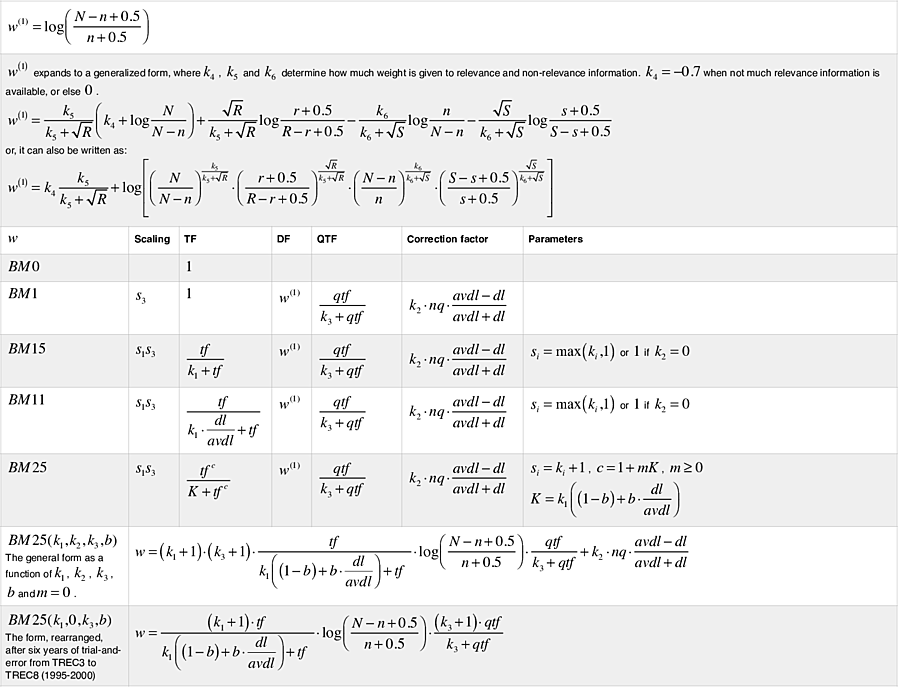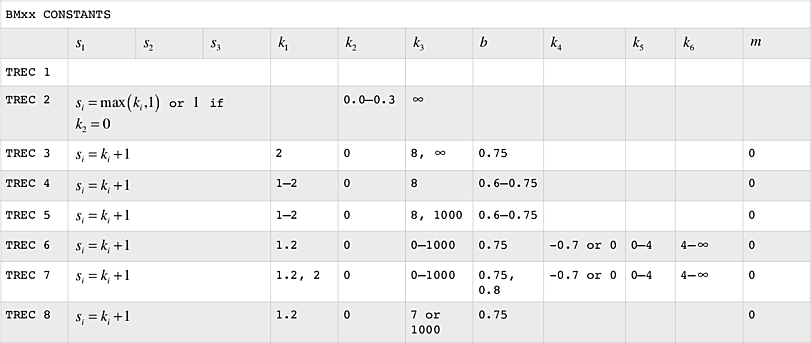#### Evolution of Okapi'S tf-idf Equations

1. Robertson SE, Jones KS. Relevance weighting of search terms. Journal of the American Society for Information science. 1976 May 1;27(3):129-46.

Puts forth arguments for using IDF; derives its form from a probabilistic model and refers to it as the F4 equation. Reasons out the use of the 0.5 in the expression.

2. Robertson SE, Walker S, Hancock-Beaulieu M, Gull A, Lau M. Okapi at TREC. InText retrieval conference 1993 Oct 1 (pp. 21-30).

Does not articulate a form of the term weighting equation but mentions that only IDF weights were used, referred to as w(1), (previously known as F4).

3. This paper is the genesis of BM25. Section 2.1 demonstrates how a formal 2-Poisson model was collapsed into a more intuitive, empirical weighting scheme, sometimes referred to as the 'rough model'. The section also says that the reasons for the term-weighting equation being chosen to be so "is not by any stretch of the imagination a strong quantitative argument; one may have many reservations about the 2-Poisson model itself, and the transformation sketched above are hardly justifiable in any formal way".

BM0, BM1, BM11, BM15 are introduced and experiments demonstrate how values of constants are found by trial and error. The report also notes; "The drawback of these two models [BM11 and BM15] is that the theory says nothing about the estimation of the constants, or rather parameters, k1 and k2. It may be assumed that these depend on the database [document collection], and probably also on the nature of the queries and on the amount of relevance information available. We do not know how sensitive they are to any of these factors. Estimation cannot be done without sets of queries and relevance judgments, and even then, since the models are not linear, they do not lend themselves to estimation by linear regression. The values we used were arrived at by long sequences of trials mainly using topics 51-100 on disks 1 and 2 database, with the TREC 1 relevance sets."

Reasoning for chosing a form for tf(): The term weighting equation formally derived from a 2-Poisson model, as a function of the term-frequency, tf(f), can be shown to behave as follows: it is zero for f = 0; it increases monotonically with f, but at an ever-decreasing rate; it approaches an asymptotic maximum as f gets large. The form tf / (tf + k) exhibits similar behavior; it has an asymptotic limit of unity.

Excerpts from the paper explaining the constants;

On k1 the author notes, "The constant k1 in the formula [BM11-TF] is not in any way determined by argument. The effect of choice of constant is to determine the strength of the relationship between weight and tf : a large constant will make for a relation close to proportionality (where tf is relatively small); a small k1 will mean that tf has relatively little effect on the weight..."

On k2 the author notes, "Values in the range 0.0—0.3 appear about right for the TREC databases (if natural logarithm is used in the term-weighting functions), with lower values being better for equation 4 [BM11-TF] termweights and the higher values for equation 3 [BM15-TF]."

On k3 the author notes, "...experiments suggest a large value of k3 to be effective--indeed the limiting case, which is equivalent to w = qtf * w(1) appears to be the most effective."

4. Robertson, S.E., Walker, S. and Hancock-Beaulieu, M.M.,1995. Large test collection experiments on an operational, interactive system: Okapi at TREC. Information Processing & Management, 31(3), pp.345-360.

Concentrates on the later TREC-2 work, meant to appear in TREC-3. Section 7 has interesting conclusions about chosing values for the constants and the nature of the curve of the term-frequency transformation. Some of the observations were:

Term-frequency should be relative and non-linear.
The document length correction factor isn't useful if term-frequency is normalized.
Query-term-frequency is useful and should be linear.

5. Robertson SE, Walker S, Jones S, Hancock-Beaulieu MM, Gatford M. Okapi at TREC-3. NIST SPECIAL PUBLICATION SP. 1995 Apr;109:109.

The BM25 weighting equation appears in its entirety in this paper. The reasons for its form, the choice of the constant c (appears as an exponent) is discussed. When concluding, in Section 9.1, the author says something interesting about the nature of IR experimentation and the role of theory: "While the field of IR remains strongly empirically driven (a tendency reinforced by the entire TREC program) ... it remains possible for an effective system design to be guided by a single theoretical framework." Section 9.3 states: "In common with most other TREC participants, we have done much too little work on analysing individual or selected groups of instances (topics, documents, terms), to try to understand in more details the circumstances under which our methods work or do not work." In the years to come this would guide the approach to IR experiments and also inspire researchers to find ways to set IR in theoretical frameworks.

6. Robertson SE, Walker S, Beaulieu MM, Gatford M, Payne A. Okapi at TREC-4. NIST SPECIAL PUBLICATION SP. 1996 Nov:73-96.

7. Beaulieu M, Gatford M, Huang X, Robertson S, Walker S, Williams P. Okapi at TREC-5. NIST SPECIAL PUBLICATION SP. 1997 Jan 31:143-66.

Section 4.3, Query Expansion says, k3 was 8 or 1000 and varying within this range made little difference.

8. Walker S, Robertson SE, Boughanem M, Jones GJ, Jones KS. Okapi at TREC-6 Automatic ad hoc, VLC, routing, filtering and QSDR. InTREC 1997 Nov 19 (pp. 125-136).

w(1) expands to a generalized form, where, k4, k5 and k6 determine how much weight is given to relevance and non-relevance information. k4 = -0.7 when not much relevance information is available, or else 0.

9. Robertson SE, Walker S, Beaulieu M, Willett P. Okapi at TREC-7: automatic ad hoc, filtering, VLC and interactive track. Nist Special Publication SP. 1999 Nov:253-64.

BM25(1.2,0,0–1000,0.75) was used for ‘adhoc’ experiments (Table 2) and BM25(2,0,1000,0.8) was used for ‘adaptive filtering’ experiments (Sec 5.6).

10. Robertson SE, Walker S. Okapi/Keenbow at TREC-8. InTREC 1999 Nov 17 (Vol. 8, pp. 151-162).

Smaller b is sometimes advantageous and large k3 is useful in long queries.

11. Robertson, S.E., Walker, S. and Beaulieu, M.,2000. Experimentation as a way of life: Okapi at TREC. Information processing & management, 36(1), pp.95-108.

One more summary of the trials, tribulations and purposes of experimentation using the Okapi system. The author points out in Section 2.2 the BM25 formula and comments that "this BM25 formula has remained more-or-less fixed since TREC-3"

## Other Papers

1. Harman D, Candela G. Retrieving records from a gigabyte of text on a minicomputer using statistical ranking. Journal of the American Society for Information Science. 1990 Dec 1;41(8):581.

2. Robertson SE, Jones KS. Simple, proven approaches to text retrieval.

3. Broglio J, Callan JP, Croft WB, Nachbar DW. Document retrieval and routing using the INQUERY system. NIST SPECIAL PUBLICATION SP. 1995:29-.